The TSCSREG Procedure
 Estimation Techniques

If the effects are fixed, the models are essentially regression models with dummy variables that correspond to the specified effects. For fixed-effects models, ordinary least squares (OLS) estimation is equivalent to best linear unbiased estimation.

The output from TSCSREG is identical to what one would obtain from creating dummy variables to represent the cross-sectional and time (fixed) effects. The output is presented in this manner to facilitate comparisons to the least squares dummy variables estimator (LSDV). As such, the inclusion of a intercept term implies that one dummy variable must be dropped. The actual estimation of the fixed-effects models is not LSDV. LSDV is much too cumbersome to implement. Instead, TSCSREG operates in a two step fashion. In the first step, the following occurs:

• One-way fixed-effects model: In the one-way fixed-effects model, the data is transformed by removing the cross-sectional means from the dependent and independent variables. The following is true: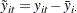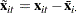• Two-way fixed-effects model: In the two-way fixed-effects model, the data is transformed by removing the cross-sectional and time means and adding back the overall means: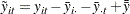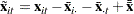where the symbols: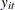and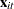are the dependent variable (a scalar) and the explanatory variables (a vector whose columns are the explanatory variables not including a constant), respectively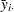and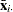are cross section means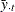and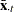are time means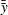and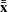are the overall means

The second step consists of running OLS on the properly demeaned series, provided that the data are balanced. The unbalanced case is slightly more difficult, because the structure of the missing data must be retained. For this case, PROC TSCSREG uses a slight specialization on Wansbeek and Kapteyn.

The other alternative is to assume that the effects are random. In the one-way case,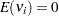,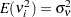, and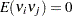for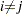, and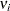is uncorrelated with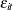for alland. In the two-way case, in addition to all of the preceding,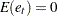,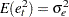, and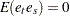for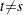, and the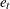are uncorrelated with theand thefor alland. Thus, the model is a variance components model, with the variance components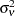,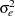, and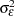, to be estimated. A crucial implication of such a specification is that the effects are independent of the regressors. For random-effects models, the estimation method is an estimated generalized least squares (EGLS) procedure that involves estimating the variance components in the first stage and using the estimated variance covariance matrix thus obtained to apply generalized least squares (GLS) to the data.Previous Page | Next Page | Top of Page